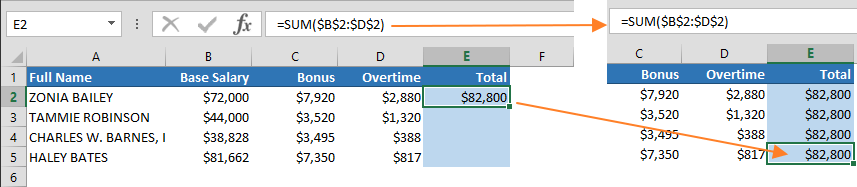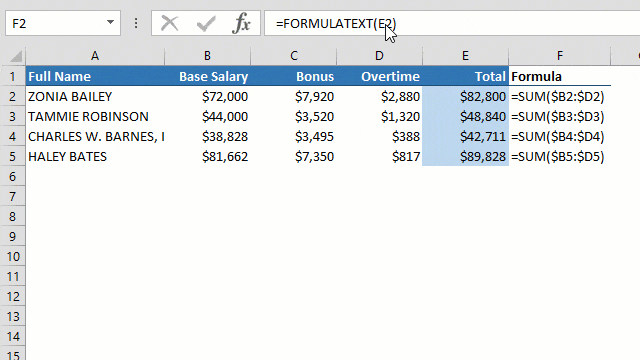Reference types can be used in Excel to determine how Excel will treat a specific target reference. By default, all references are relative references, and this means that all subsequent actions will be relative to the position of that reference. To switch into an Excel absolute reference, you must specify this by adding a special character into that reference. In this article, we’re going to show you how to create Excel absolute references and relative references using the dollar (\$) sign.

## Excel Absolute Reference and Relative Reference

Excel uses three terms when defining cell or range references:

• Absolute
• Relative
• Mixed

These two types of references come into play when copying and auto-filling cells. When copying a cell or range that contains formulas, absolute references in the formulas will remain same. On the other hand, relative references will be updated automatically, base on where the cell or range is copied.

For example; assume that cell E2 below contains the formula =SUM(B2:D2) and you want to copy it down through cells E3 and E5.After copying, you will see that the references in the formulas are different than the formula in the originating cell E2. E3 gets =SUM(B3:D3), E4 gets =SUM(B4:D4) and finally E5 gets =SUM(B5:D5).The references are updated because the originating cell E2 uses relative references. If an Excel absolute reference was to be used, the cell reference would remain the same as it appears in the formula from the source cell. The screenshot below shows using absolute references instead.A mixed reference is a mixed use of both absolute and relative references. For example; we can use a mixed reference that will lock the column because we do not need to update the column when copying a formula down to the rest of the column. \$B2:\$D2 is a range where columns are absolute and rows are relative.## Switching between relative, absolute, and mixed references

As you can see, visual difference between reference is a dollar sign (\$). The "\$" essentially locks the column or row identifier in front of it. The locked identifier won't change when you copy that reference.

There are two ways to add the \$ sign to create absolute, relative and mixed references:

1. Typing in \$ manually where applicable
2. Pressing F4 (Windows), or CMD + T (Mac)

You can manually enter the \$ sign to make a reference absolute. Alternatively, when reference is selected or the cursor is near the reference text, pressing the F4 key will switch between references quickly.## Summary

 \$A1 Column remains the same, row gets updated A\$1 Row remains the same, column gets updated \$A\$1 Both row and column remains the same